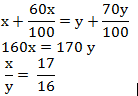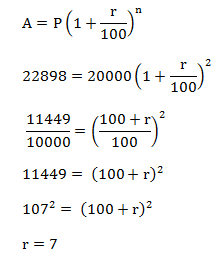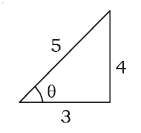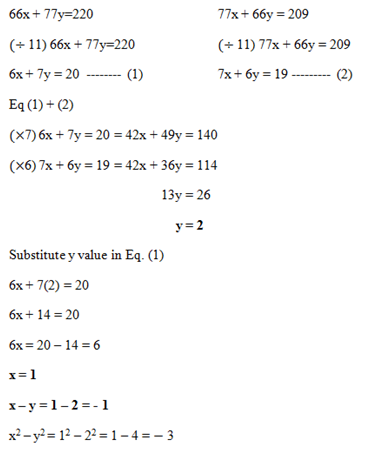# SSC CGL 2018 Practice Test Papers | Quantitative Aptitude (Day-53)

Dear Aspirants, Here we have given the Important SSC CGL Exam 2018 Practice Test Papers. Candidates those who are preparing for SSC CGL 2018 can practice these questions to get more confidence to Crack SSC CGL 2018 Examination.

[WpProQuiz 3615]

Click “Start Quiz” to attend these Questions and view Explanation

1) Salary of Aswini is equal to the salary of Banu. But their basic pay is different. The allowances are 60% and 70% of the basic pay respectively. What is the ratio of the basic pay of Aswini to Banu?

a) 12: 15

b) 17: 16

c) 13: 19

d) 7: 6

2) A man went for trekking and climbing up a mountain of 240 meters height. He climbs 60 m in a day but slipped back by 12 m and 15 cm in the evening. How far had the man reached by end of the third day?

a) 122

b) 143.55

c) 158. 22

d) 164. 75

3) In a textile mill, the production of sarees increases to 22,898 from 20,000 in 2 years. What is the rate of growth of production per annum?

a) 10%

b) 10.5%

c) 9%

d) 7%

4) Z men can do a piece of work in 36 days. They worked together for 12 days, then 20 men joined them then work was completed in 16 more days .Find the total number of men employed to do work in the beginning?.

a) 46

b) 40

c) 35

d) 32

5) The length of a Rectangular plot is increased by 20%. By what percentage its corresponding breadth must be decreased to retain the original area of the plot?

a) 13 1/3%

b) 20%

c) 16 2/3%

d) 25%

6) If sin θ = 4/5, Find the value of cosec θ + cot θ.

a) 8/5

b) 2

c) 3/5

d) 7/2

7) Find the value of x.

552+133 = 722+ x.

a) 42

b) 38

c) 35

d) 43

8) A stationery shop owner buys 120 pencils for Rs.360. Some of these pencils were broken and they are left unused.  He sells the remaining pencils at Rs. 4 each and makes a profit of Rs.60. What is the % of pencils which are broken?

a) 26%

b) 12.5%

c) 15%

d) 18%

9) Ankul marks his goods 30% above cost price and provides a discount of 10%, Find the cost price of a tea pot which is sold for Rs.234.

a) Rs. 196

b) Rs. 180

c) Rs. 200

d) Rs. 108

10) 66x + 77y=220 and 77x + 66y = 209. What is the value of x2 – y2 if x – y = – 1?

a) – 2

b) 3

c) – 3

d) – 1Distance covered in a day = 60 – 12.15 = 47.85

Distance covered by the end of third day = 47.85×3 = 143.55

The number of sarees after 2 years

= 22898

The number of sarees in present year

= 20000

Let, the percentage growth of companym1×d1= m2 ×d2

Z×36 = Z×12+ (Z+20) ×16

36Z = 12Z+ 16Z + 320

36Z – 28Z = 320

8Z = 320

Z = 40

Number of men employed in the beginning = 40

Length = x

Length increased by 20 % = 120/100 x length = 6x/5

To have equal area breadth should be multiplied by reciprocal of 120/100

Percentage decrease = (b – 100b/120)/ b x100

= 20b/120b x100

= 16 2/3 %

(or)

To retain the area of rectangle the breadth should be decreased by

= (20×100)/(100 + 20)

= 2000 / 120

= 16 2/3 %Sin θ = opposite side / hypotenuse = 4/5

Cosec θ = 1/sin θ = 5/4

Tan θ = opposite side / adjacent side = 4/3

Cot θ = 1/ tan θ =3/4

Cosec θ+ cot θ= 5/4 + ¾ = 8/4 =2

552 + 133 = 722 + x

X = 3025 + 2197 – 5184

X = 38

Let the number of Pencils broken be x.

Cost Price of 120 Pencils =360

Cost Price of a pencil = 360/120 =Rs. 3

Selling Price of a Pencil = Rs. 4

Profit in selling 1 Pencil = 4 – 3 = 1

Total Profit = 60

(120 – x) (1) – 3x = 60

120 – x – 3x = 60

4x = 60

X = 15

Number of Pencils broken = 15

Percentage of Pencils broken = 15/120×100 = 12.5%

Let the cost price be x. Marked Price = 130/100 × x

Selling Price = 90/100 ×130/100 × x = 234

X = 200

Cost Price = Rs. 200